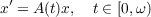#### Vol. 33, No. 3, 1970

 Download this articleFor screen For printingRecent Issues Vol. 325: 1  2 Vol. 324: 1  2 Vol. 323: 1  2 Vol. 322: 1  2 Vol. 321: 1  2 Vol. 320: 1  2 Vol. 319: 1  2 Vol. 318: 1  2Online Archive Volume: Issue:The Journal Subscriptions Editorial Board Officers Contacts Submission Guidelines Submission Form Policies for Authors ISSN: 1945-5844 (e-only) ISSN: 0030-8730 (print) Special Issues Author Index To Appear Other MSP Journals
The asymptotic behaviour of solutions to linear systems of ordinary differential equations

### Jack W. Macki and James Stephen Muldowney

Vol. 33 (1970), No. 3, 693–706
##### Abstract

This paper is concerned with the system of differential equations(1)

where A(t) is an n×n matrix of locally integrable complexvalued functions on [0) and x(t) is an n-dimensional column vector. The class of matrices A(t) such that (1) has a nontrivial solution x0(t) satisfying limtω|x0(t)| = 0 is denoted by Ω0; the class of matrices A(t) such that (1) has a solution x(t) satisfying limtω|x(t)| = +is denoted by Ω. If P is a projection then ΩP0 denotes the class of matrices A(t) such that (1) has a nontrivial solution x0(t) satisfying limtω|Px0(t)| = 0. Sufficient conditions are given for A(t) Ω0,A(t) Ω and A(t) ΩP0; the result, obtained include as special cases theorems of Coppel, Hartman, and Milloux.

Primary: 34.50
##### Milestones